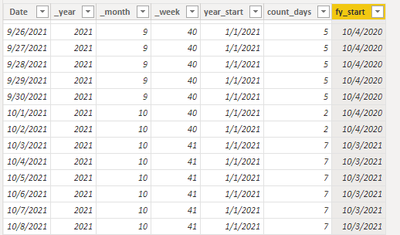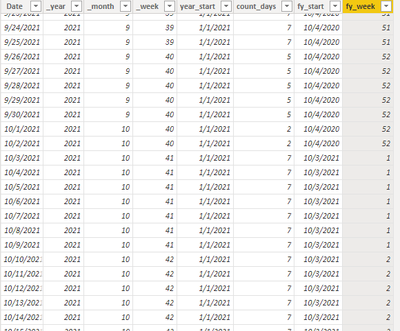cancel
Showing results for
Did you mean:## Custom Fiscal Week

Scenario:

Fiscal years/months/weeks are often mentioned when our calculations involve dates. However, a common calendar table cannot meet such needs. At this time, we need to create a fiscal calendar table. Certainly, it is relatively simple to create a fiscal calendar table by month or by week, and there are already many blogs about it. Therefore, I would like to share with you how to designate the first full week of a specific month as the start of the fiscal year.

Preparation:

Operations:

Step1: Add year, month, week and start of year column to the calendar table.

``_year = YEAR('calendar'[Date])``
``_month = MONTH('calendar'[Date])``
``_week = WEEKNUM('calendar'[Date])``
``year_start = STARTOFYEAR('calendar'[Date])``

Step2: Find the first full week of October.

``````count_days =

CALCULATE (

COUNT ( 'calendar'[Date] ),

FILTER (

'calendar',

'calendar'[_year] = EARLIER ( 'calendar'[_year] )

&& 'calendar'[_month] = EARLIER ( 'calendar'[_month] )

&& 'calendar'[_week] = EARLIER ( 'calendar'[_week] )

)

)``````Step3: Add the conditions -- the first full week of October into the formula to get the start date of the fiscal year.

``````fy_start =

CALCULATE (

MIN ( 'calendar'[Date] ),

FILTER (

'calendar',

'calendar'[_year] = EARLIER ( 'calendar'[_year] )

&& 'calendar'[_month] = 10

&& 'calendar'[count_days] = 7

)

)``````

Here we will find a problem that in our logic, dates before October 3, 2021 are the dates of the previous fiscal year, so the start date of the fiscal year is incorrect.So we need to adjust our formula and add an if condition.

``````fy_start =

VAR this_year =

CALCULATE (

MIN ( 'calendar'[Date] ),

FILTER (

'calendar',

'calendar'[_year] = EARLIER ( 'calendar'[_year] )

&& 'calendar'[_month] = 10

&& 'calendar'[count_days] = 7

)

)

VAR _DYNAMIC_DimDate =

CALENDAR (

DATE ( 'calendar'[_year] - 1, 10, 1 ),

DATE ( 'calendar'[_year] - 1, 10, 31 )

),

"Year", YEAR ( [Date] ),

"Month", MONTH ( [Date] ),

"Weeknum", WEEKNUM ( [Date] )

)

_DYNAMIC_DimDate,

"Count",

COUNTAX (

FILTER (

_DYNAMIC_DimDate,

[Year] = EARLIER ( [Year] )

&& [Month] = EARLIER ( [Month] )

&& [Weeknum] = EARLIER ( [Weeknum] )

),

[Date]

)

)

VAR last_year =

MINX ( FILTER ( _ADD, [Count] = 7 ), [Date] )

RETURN

IF ( 'calendar'[Date] >= this_year, this_year, last_year )``````

Now the result is correct.At this point we have the start date of the fiscal year under the specified conditions, and our main work is done.

Step4: As a final step, we will customize the week number with this start date.

``````fy_week =

ROUNDUP (

( DATEDIFF ( 'calendar'[fy_start], 'calendar'[Date], DAY ) + 1 ) / 7,

0

)``````Summary:

First we need to convert the conditions to DAX formula -- the first full week of October (count = 7). Then we use the conditions to get the start date of a fiscal year. Since the fiscal year and the calendar do not exactly coincide, we need to consider the fiscal year that each date corresponds to. At last, we add a custom fiscal week with the start date of the fiscal year.